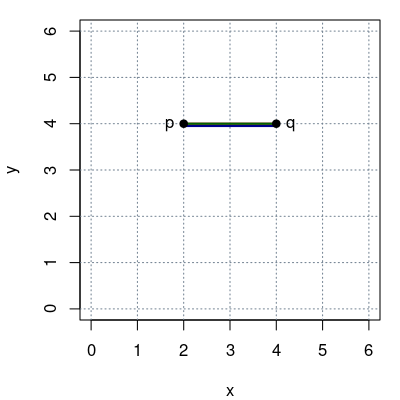## Exam 1

1. #### Question

Given two points $p=\left(2,4\right)$ and $q=\left(4,4\right)$ in a Cartesian coordinate system:
1. What is the Manhattan distance ${d}_{1}\left(p,q\right)$?
2. What is the Euclidean distance ${d}_{2}\left(p,q\right)$?
3. What is the maximum distance ${d}_{\mathit{\infty }}\left(p,q\right)$?

#### Solution

The distances are visualized below in green (${d}_{1}$), red (${d}_{2}$), and blue (${d}_{\mathit{\infty }}$).1. ${d}_{1}\left(p,q\right)=\sum _{i}|{p}_{i}-{q}_{i}|=|2-4|+|4-4|=2$.
2. ${d}_{2}\left(p,q\right)=\sqrt{\sum _{i}\left({p}_{i}-{q}_{i}{\right)}^{2}}=\sqrt{\left(2-4{\right)}^{2}+\left(4-4{\right)}^{2}}=2$.
3. ${d}_{\mathit{\infty }}\left(p,q\right)=\underset{i}{max}|{p}_{i}-{q}_{i}|=max\left(|2-4|,|4-4|\right)=2$.